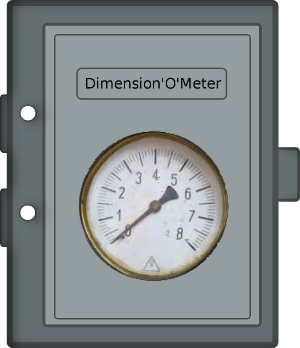# How many dimensions does our world have?The Dimension-O-Meter

Let’s build a special machine: a Dimension-O-Meter. This is a sort of box (apparently so), with a button on the top and a dial on the front, and a big needle indicating some numbers: 1, 2, 3, 4… When I press the button, the machine start buzzing loudly, indicating it is making all sorts of measurements and calculations. The needle then takes off and slowly stabilises on a number: 3. Of course, if run in a different Universe, this machine could indicate a different number… How could we build such a machine?

### The degrees of liberty

Let’s see… What does define the number of dimensions? It is said that our Universe have three (observable) dimensions. Those dimensions seems to be related to the way I define the position of an object. How many numbers do I need to define precisely the position of an object? Three? Wrong, the answer is six. With only three numbers for translations, the object can still rotate on itself so its position is not entierely defined. Why does a space in three dimensions authorizes three translations and three rotations? Let’s try to see what happens in other dimensions:

• In dimension one (moving along a line), there is no rotation.
• In dimension two (moving on a plane), there is one rotation: rotating around a point.
• In dimension three, there are three rotations.
• In dimension four, there are… six rotations.

This is summed up in the following table:

Dimensions Translations Rotations
1 1 0
2 2 1
3 3 3
4 4 6
n n n*(n-1)/2

This site gives a lot of informations about rotations in n dimensions. So our machine could try to rotate an object and see how many possible rotations there are.

### The scaling

Another way to determine the number of dimensions is to try to scale an object. In dimension one, if I take an object (a segment) and I scale it up by a factor of two, its apparent size (the length) is doubled. In dimension two, if I double the scale of an object (a square), its size (the area) is multiplied by four. In dimension three, if I double the scale of an object (a cube), its size (the volume) is multiplied by eight. Is there a relation?Relation between scaling, size and dimensions

The relation seems to be the following:

$$N = l^D$$

Where D is the dimension, l is the scaling factor (doubling is our exemple) and N is the measure of the size (length, area, volume) of the resulting object. D is called the Hausdorff dimension. We thus obtain:

$$D = \frac{\log N}{\log l}$$

So our machine could also try to scale up an object and see by how much it inflates. Interestingly, this relation extends to non-integer dimensions for fractals, as shown in this article.

### The Simplex

Yet another method is to try to draw a simplex. A simplex is the generalization of an triangle to n dimensions. It is thus a tetrahedron in dimension 3.a 3 dimensional simplex

If you can draw a regular simplex with $$n$$ vertices, then you are in dimension $$n - 1$$.

### Conclusion

To say the truth, this machine will probably be difficult to build… The main reason is more phylosophical than technical: the number of dimensions is not a measure. It is not a measure because measuring something implies to define an isolated sub-system that we want to measure. The measurement tools (a ruler, for example) should be outside the system. This is the difficulty with the dimensions: as they encompass the whole Universe, in some sense, I cannot place myself outside of the Universe to measure it.